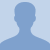# Konst Math: Adding and Subtracting FractionsLike fractions are fractions with the same denominator. You can add and subtract like fractions easily - simply add or subtract the numerators and write the sum over the common denominator. Before you can add or... Like fractions are fractions with the same denominator. You can add and subtract like fractions easily - simply add or subtract the numerators and write the sum over the common denominator. Before you can add or subtract fractions with different denominators, you must first find equivalent fractions with the same denominator, like this: Find the smallest multiple (LCM) of both numbers. Rewrite the fractions as equivalent fractions with the LCM as the denominator.
More... Collapse
134 Views

## Comments (0)

Please log in to post comments.